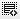## calculating salary percentage?1
Video no:6 on advanced income statement there is question to calculate % growth of salary expenses between 2008 and 2009. I have managed to get the right answer but I am totally confused with the answer. can you please elaborate on the same. on top, I am little confused with the basics of calculation of percentage so can you please explain0

Two things important to answer the question:
1. Which data to take.
2. Calculate the Growth%.

Financial year for any company is from April 1, last year to March 31, current year.

So, the expenses for both the years are taken for the March 31 of that particular year.

Calculating Growth rate = [(t+1)value - (t)value]*100/[(t)value]

Error in approximation may also lead to incorrect answer as the options given are very close to the answer.0

They have just asked what has been the increase in terms of percentage in the case of the expense Salary.
Suppose the total salary expense was Rs.40000 and Rs.50000 for the year 2008 and 2009 respectively and if you were asked what has been the increase the answer to it would be pretty straight forward i.e Rs.10000
We have been asked to find the same, the only difference being the answer needs to be in terms of percentage. Now a Rs.10000 increase is nothing but an increase of 25%, calculated as follows:
(50000-40000)/40000 * 100 = 25%

Formula: Ending value - Beginning value/Beginning value * 100
2009 yr amt. 2008 yr amt.

I am not referring to the actual income statement and assuming the numbers here as it was getting a bit tedious to pull out the actual statement. Also i hope you were facing a problem with just the calculation part so this should suffice.0

In the Dominos pizza income statement, the salary is given through the line item "Staff Costs"

Click on thisicon to add code snippet.